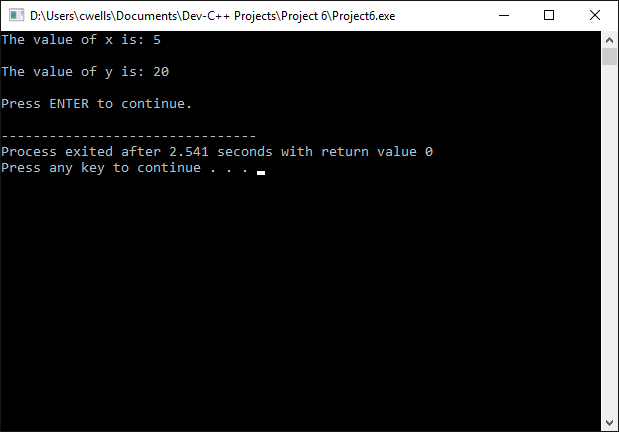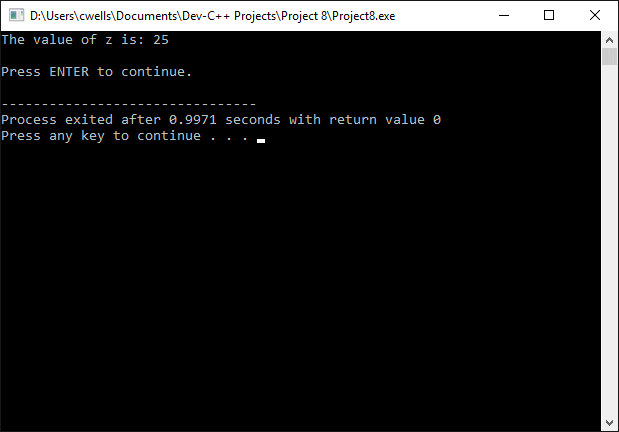# C++ Operators

The assignment operator (=) assigns a value to a variable, as shown below.

x = 25;

This statement assigns a value of 25 to the variable x. The term to the left of the operator is known as the lvalue (left value), and the term to the right of the operator is known as the rvalue (right value). The lvalue must be a variable, but the rvalue can be a constant, another variable, or an expression. Assignment is always carried out from right to left (the value of rvalue is assigned to lvalue). Any value stored in lvalue before the assignment takes place is replaced by the value in rvalue. The following short program demonstrates the use of the assignment operator.

// Example Program 1

#include <iostream>
using namespace std;

#define PI 3.14159265

int main ()
{
string s;
int x, y;  // value of x and y is undetermined

x = 10;    // x is assigned a value of 10
y = 5;     // y is assigned a value of 5
x = y;     // the value of y (5) is assigned to x
y = 20;    // y is assigned a value of 20
cout << "The value of x is: ";
cout << x;
cout << "\n\n";
cout << "The value of y is: ";
cout << y;
cout << "\n\nPress ENTER to continue.";
getline( cin, s );
return 0;
}The output from example program 1

## Arithmetic operators

The basic arithmetic operators provided by C++ are shown in the table below.

The C++ arithmetic operators
SymbolPurpose
-subtraction
*multiplication
/division
%modulo

Most of these arithmetic operators will be familiar to you. The modulo operator returns the remainder of a division operation. For example, the following statement assign a value of 3 to x:

x = 15 % 6

Fifteen divided by six gives a result of two remainder three. The remainder is assigned to the lvalue when the modulo operator is used, so x becomes three.

## Compound assignment operators

We can modify the value of a variable by performing an arithmetic operation on the value already stored in that variable using compound assignment operators. The compound assignment operators provided by C++ are listed below.

The C++ compound assignment operators
SymbolExampleMeans the same as
+=x += yx = x + y
-=x -= yx = x - y
*=x *= yx = x * y
/=x /= yx = x / y
%=x %= yx = x % y
>>=x >>= yx = x >> y
<<=x <<= yx = x << y
&=x &= yx = x & y
^=x ^= yx = x ^ y
|=x |= yx = x | y

The short program below demonstrates the use of the += operator.

// Example Program 2

#include <iostream>
using namespace std;

int main ()
{
int x = 25;
string s;

x += 10;  // equivalent to x = x + 10
cout << "The value of x is: ";
cout << x;
cout << "\n\nPress ENTER to continue.";
getline( cin, s );
return 0;
}The output from example program 2

## Increment and decrement operators

The increment operator (++) increases the value of the variable to which it is applied by one. The following statements are equivalent to one another:

x++;
x = x + 1;

The decrement operator (--) decreases the value of the variable to which it is applied by one. The following statements are equivalent to one another:

x--;
x = x - 1;

Both the increment and decrement operators can be used as a prefix or as a suffix to a variable. In the above examples these operators are used as a suffix, i.e. they follow the variable. Although the effect on the variable itself will be exactly the same in either case, the value of the variable when used in a more complex expression may differ, depending on whether the operator comes before or after the variable. The following code fragments illustrate this point.

x = 10;
y = ++x;
// the value of x becomes 11, and the value assigned to y is 11

x = 10;
y = x++;
// the value of x becomes 11, but the value assigned to y is 10

## Comparison operators

The comparison operators compare the values of two variables or expressions and return a Boolean value (true or false), depending on the result of the comparison. The C++ comparison operators are described in the table below.

The C++ comparison operators
SymbolMeaning
==Equal to
!=Not equal to
>Greater than
<Less than
>=Greater than or equal to
<=Less than or equal to

Below are some examples that use these operators.

10 == 20   // evaluates to false
10 != 15   // evaluates to true
10 > 3     // evaluates to true
5 < 4      // evaluates to false
25 >= 25   // evaluates to true
25 <= 24   // evaluates to false

As well as numeric constants, we could use any valid expression or variable. Note that the assignment operator (=) has a completely different function to that of the equality operator (==).

## Logical operators

The logical NOT operator (!) negates the operand located immediately to its right, returning false if the operand is true, and true if the operand is false. The following code fragments illustrate how this operator works.

!(25 == 25)  // evaluates to false
!(26 <= 24)  // evaluates to true
!true        // evaluates to false
!false       // evaluates to true

The logical AND operator (&&) returns true if both its operands are true, and false otherwise. The following truth table shows the possible outcomes.

The C++ AND (&&) operator
xyx && y
truetruetrue
truefalsefalse
falsetruefalse
falsefalsefalse

The logical OR operator (||) returns true if either one of its operands is true, and false only if both operands are false. The following truth table shows the possible outcomes.

The C++ OR (||) operator
xyx || y
truetruetrue
truefalsetrue
falsetruetrue
falsefalsefalse

## The conditional operator

The conditional operator (?) evaluates an expression and returns one of two possible values, depending on whether the expression evaluates to true or false. The general syntax is shown below.

condition ? option1 : option2;

If the condition evaluates to true, the expression returns option1, otherwise it returns option2. The following short program illustrates the use of this operator.

// Example Program 3

#include <iostream>
using namespace std;

int main ()
{
int x, y, z;
string s;

x = 10;
y = 25;
z = (x > y) ? x : y;
cout << "The value of z is: ";
cout << z;  // outputs a value for z of 25 (y)
cout << "\n\nPress ENTER to continue.";
getline( cin, s );
return 0;
}The output from example program 3

In the above example, the expression x > y was false, so z was assigned the value of the second option (y).

## The comma operator

The comma operator (,) separates two or more expressions that are evaluated in sequence. The result of evaluating the final expression in the sequence is then assigned to the lvalue. The following code fragment demonstrates the use of the comma operator.

x = (y = 10, y + 5);

The above statement would assign a value of 15 to x.

## Bitwise operators

Bitwise operators modify variables according to the binary bit patterns that represent the values they store.

The C++ bitwise operators
OperatorDescription
&Bitwise AND
|Bitwise Inclusive OR
^Bitwise Exclusive OR
~One's complement (bit inversion)
<<Shift left
>>Shift right

## Operator precedence

Operator precedence determines which elements in a complex expression are evaluated first. C++ organises all operators into the priority order shown in the following table.

C++ operator precedence
LevelOperatorDescriptionAssociativity
1::Scope resolutionnone
2( )Grouping operatorleft-to-right
[ ]Array access
->Member access from a pointer
.Member access from an object
++Post-increment
--Post-decrement
3!Logical negationright-to-left
~Bitwise complement
++Pre-increment
--Pre-decrement
-Unary minus
+Unary plus
*Dereference
(type)Cast to a given type
sizeofReturn size in bytes
4->*Member pointer selectorleft-to-right
.*Member object selector
5*Multiplicationleft-to-right
/Division
%Modulus
-Subtraction
7<<Bitwise shift leftleft-to-right
>>Bitwise shift right
8<Comparison less-thanleft-to-right
<=Comparison less-than-or-equal-to
>Comparison greater-than
>=Comparison greater-than-or-equal-to
9==Comparison equal-toleft-to-right
!=Comparison not-equal-to
10&Bitwise ANDleft-to-right
11^Bitwise exclusive ORleft-to-right
12|Bitwise inclusive (normal) ORleft-to-right
13&&Logical ANDleft-to-right
14||Logical ORleft-to-right
15? :Ternary conditional (if-then-else)right-to-left
16=Assignment operatorright-to-left
+=Increment and assign
-=Decrement and assign
*=Multiply and assign
/=Divide and assign
%=Modulo and assign
&=Bitwise AND and assign
^=Bitwise exclusive OR and assign
|=Bitwise inclusive (normal) OR and assign
<<=Bitwise shift left and assign
>>=Bitwise shift right and assign
17,Sequential evaluation operatorleft-to-right

The grouping operator (parentheses) can be used to force the expression contained within the brackets to be evaluated first, irrespective of the precedence of any other operators in a statement outside the parentheses.